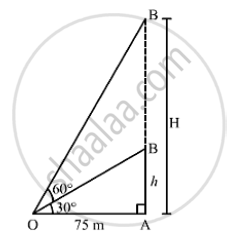# The Angle of Elevation of the Top of an Unfinished Tower a T a Distance of 75m from Its Base is 30° . How Much Higher Must the Tower Be Raised So that the Angle of Elevation of Its Top at Same Point. - Mathematics

The angle of elevation of the top of an unfinished tower at a distance of 75m from its base is 30° .How much higher must the tower be raised so that the angle of elevation of its top at the same point may be 60 .

#### Solution

Let AB be the unfinished tower, AC be the raised tower and O be the point of observation
We have:
OA = 75m,∠AOB = 30°  and ∠AOC = 60°
Let AC = H m such that BC = (H -h)m.In ΔAOB,we have:
(AB)/(OA) = tan 30° = 1/ sqrt(3)

⇒ h/ 75 = 1/ sqrt(3)

⇒ = 75/ sqrt(3) m = (75 xx sqrt(3)) /(sqrt(3) xx sqrt(3)) =  25 sqrt(3) m
In ΔAOC,we have:
(AC)/(OA) = tan 60° = sqrt(3)

⇒ H/75 = sqrt(3)

⇒ H = 75 sqrt(3 )m

∴"Required height" =(H - h) = (75 sqrt(3) - 25 sqrt(3)) = 50 sqrt(3)m = 86.6m

Concept: Heights and Distances
Is there an error in this question or solution?
Chapter 14: Height and Distance - Exercises

#### APPEARS IN

RS Aggarwal Secondary School Class 10 Maths
Chapter 14 Height and Distance
Exercises | Q 9

Share